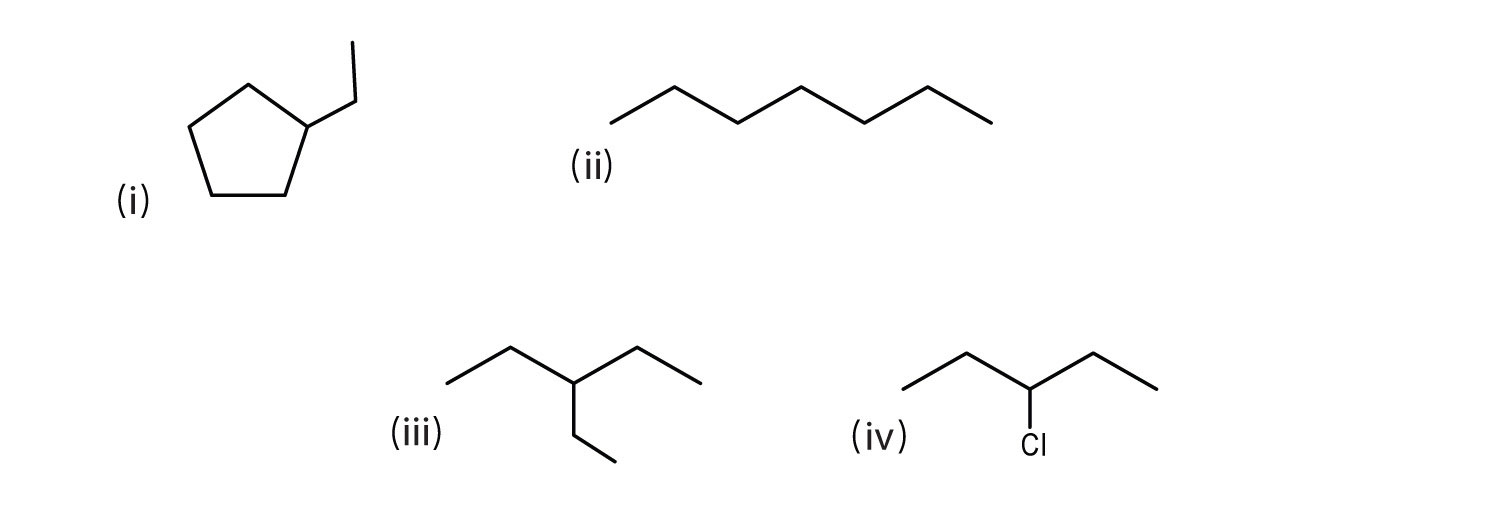## 12.10 End-of-Chapter Material

### Chapter Summary

To ensure that you understand the material in this chapter, you should review the meanings of the following bold terms in the summary and ask yourself how they relate to the topics in the chapter.

Organic chemistry is the chemistry of carbon compounds, and inorganic chemistry is the chemistry of all the other elements. Carbon atoms can form stable covalent bonds with other carbon atoms and with atoms of other elements, and this property allows the formation the tens of millions of organic compounds. Hydrocarbons contain only hydrogen and carbon atoms.

Hydrocarbons in which each carbon atom is bonded to four other atoms are called alkanes or saturated hydrocarbons. They have the general formula CnH2n + 2. Any given alkane differs from the next one in a series by a CH2 unit. Any family of compounds in which adjacent members differ from each other by a definite factor is called a homologous series.

Carbon atoms in alkanes can form straight chains or branched chains. Two or more compounds having the same molecular formula but different structural formulas are isomers of each other. There are no isomeric forms for the three smallest alkanes; beginning with C4H10, all other alkanes have isomeric forms.

A structural formula shows all the carbon and hydrogen atoms and how they are attached to one another. A condensed structural formula shows the hydrogen atoms right next to the carbon atoms to which they are attached. A line-angle formula is a formula in which carbon atoms are implied at the corners and ends of lines. Each carbon atom is understood to be attached to enough hydrogen atoms to give each carbon atom four bonds.

The IUPAC System of Nomenclature provides rules for naming organic compounds. An alkyl group is a unit formed by removing one hydrogen atom from an alkane.

The physical properties of alkanes reflect the fact that alkane molecules are nonpolar. Alkanes are insoluble in water and less dense than water.

Alkanes are generally unreactive toward laboratory acids, bases, oxidizing agents, and reducing agents. They do burn (undergo combustion reactions).

Alkanes react with halogens by substituting one or more halogen atoms for hydrogen atoms to form halogenated hydrocarbons. An alkyl halide (haloalkane) is a compound resulting from the replacement of a hydrogen atom of an alkane with a halogen atom.

Cycloalkanes are hydrocarbons whose molecules are closed rings rather than straight or branched chains. A cyclic hydrocarbon is a hydrocarbon with a ring of carbon atoms.

1. You find an unlabeled jar containing a solid that melts at 48°C. It ignites readily and burns readily. The substance is insoluble in water and floats on the surface. Is the substance likely to be organic or inorganic?

2. Give the molecular formulas for methylcyclopentane, 2-methylpentane, and cyclohexane. Which are isomers?

3. What is wrong with each name? (Hint: first write the structure as if it were correct.) Give the correct name for each compound.

1. 2-dimethylpropane
2. 2,3,3-trimethylbutane
3. 2,4-diethylpentane
4. 3,4-dimethyl-5-propylhexane
4. What is the danger in swallowing a liquid alkane?

5. Distinguish between lighter and heavier liquid alkanes in terms of their effects on the skin.

6. Following is the line formula for an alkane. Draw its structure and give its name.7. Write equations for the complete combustion of each compound.

1. propane (a bottled gas fuel)
2. octane (a typical hydrocarbon in gasoline).
8. The density of a gasoline sample is 0.690 g/mL. On the basis of the complete combustion of octane, calculate the amount in grams of carbon dioxide (CO2) and water (H2O) formed per gallon (3.78 L) of the gasoline when used in an automobile.

9. Draw the structures for the five isomeric hexanes (C6H14). Name each by the IUPAC system.

10. Indicate whether the structures in each set represent the same compound or isomers.

1.2.3.11. Consider the line-angle formulas shown here and answer the questions.1. Which pair of formulas represents isomers? Draw each structure.
2. Which formula represents an alkyl halide? Name the compound and write its condensed structural formula.
3. Which formula represents a cyclic alkane? Name the compound and draw its structure.
4. What is the molecular formula of the compound represented by (i)?

1. organic

1. Two numbers are needed to indicate two substituents; 2,2-dimethylpropane.
2. The lowest possible numbers were not used; 2,2,3-trimethylbutane.
3. An ethyl substituent is not possible on the second carbon atom; 3,5-dimethylheptane.
4. A propyl substituent is not possible on the fifth carbon atom; 3,4,5-trimethyloctane.
2. Lighter alkanes wash away protective skin oils; heavier alkanes form a protective layer.

1. C3H8 + 5O2 → 3CO2 + 4H2O
2. 2C8H18 + 25O2 → 16CO2 + 18H2O
3. CH3CH2CH2CH2CH2CH3; hexane1. ii and iii; CH3CH2CH2CH2CH2CH2CH3 and2. iv; 3-chloropentane; CH3CH2CHClCH2CH3
3. i; ethylcyclopentane;4. C7H14